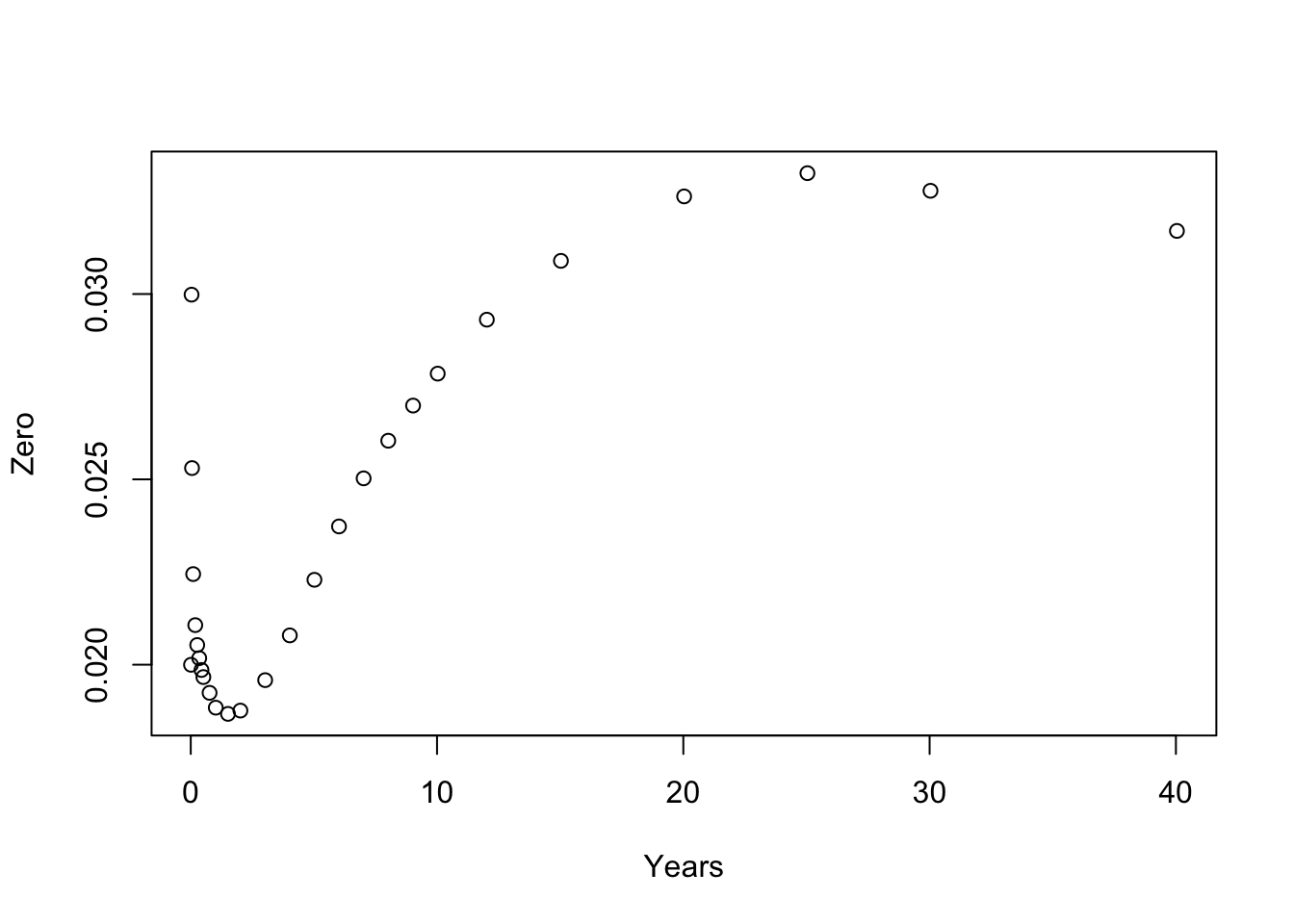Implements basic financial market objects like currencies, currency pairs, interest rates and interest rate indices. You will be able to use Benchmark instances of these objects which have been defined using their most common conventions or those defined by International Swap Dealer Association (ISDA, https://www.isda.org) legal documentation.

## Basic objects

You can create instances of key currencies and currency pairs (and of course create your own implementations):

library("fmdates")
library("fmbasics")
AUD()
#> <Currency> AUD
AUDUSD()
#> <CurrencyPair> AUDUSD

These come with implementations of handy methods:

library("lubridate")
to_fx_value(dates = ymd(20171230), tenor = "spot", x = AUDUSD())
#>  "2018-01-03"
to_fx_value(ymd(20171230), months(3), AUDUSD())
#>  "2018-04-03"

You can create instances of key IBOR or ONIA interest rate indices:

USDLIBOR(months(3))
#> <IborIndex> 3m USD LIBOR
EONIA()
#> <CashIndex> EONIA

These also come with implementations of handy methods:

to_reset(dates = as.Date("2017-01-20"), index = USDLIBOR(months(3)))
#>  "2017-01-18"
to_value(as.Date("2017-01-20"), USDLIBOR(months(3)))
#>  "2017-01-24"
to_maturity(as.Date("2017-01-20"), USDLIBOR(months(3)))
#>  "2017-04-20"

## Interest rates and discount factors

You can create and perform basic manipulation of interest rates and discount factors:

rr <- InterestRate(value = 0.01, compounding = Inf, day_basis = "act/365")
as_DiscountFactor(rr, d1 = ymd(20170120), d2 = ymd(20210120))
#> <DiscountFactor> 0.960763116514576, 2017-01-20--2021-01-20
# Convert to different rate basis
as_InterestRate(rr, day_basis = "act/360")
#> <InterestRate> 0.9863014%, CONTINUOUS, ACT/360
as_InterestRate(rr, compounding = 2, day_basis = "act/360")
#> <InterestRate> 0.9887373%, SEMI-ANNUAL, ACT/360
dd <- DiscountFactor(0.75, d1 = ymd(20170120), d2 = ymd(20210120))
as_InterestRate(dd, compounding = Inf, day_basis = "act/360")
#> <InterestRate> 7.088675%, CONTINUOUS, ACT/360

## Pricing objects

It is also possible to create and interpolate on zero coupon interest rate curves:

zc <- build_zero_curve()
plot(zc$pillar_times, zc$pillar_zeros, xlab = 'Years', ylab = 'Zero')interpolate(zc, year_frac(zc\$reference_date, ymd(20170331), "act/365"))
#>  0.01858073
interpolate_zeros(zc, ymd(20170331))
#> <InterestRate> 1.858073%, CONTINUOUS, ACT/365
interpolate_fwds(zc, ymd(20170331), ymd(20170630))
#> <InterestRate> 1.837645%, SIMPLE, ACT/365
interpolate_dfs(zc, ymd(20170331), ymd(20170630))
#> <DiscountFactor> 0.995439369532595, 2017-03-31--2017-06-30

Basic support for creating and interpolating volatility surfaces is possible:

vs <- build_vol_surface()
at <- tibble::tibble(
maturity = as.Date(c("2020-03-31", "2021-03-31")),
smile = c(0.40, 0.80)
)
interpolate(vs, at)
#>  0.6001969 0.6042421

Further details can be found in this package’s help pages and vignettes (vignette(package = "fmbasics"))